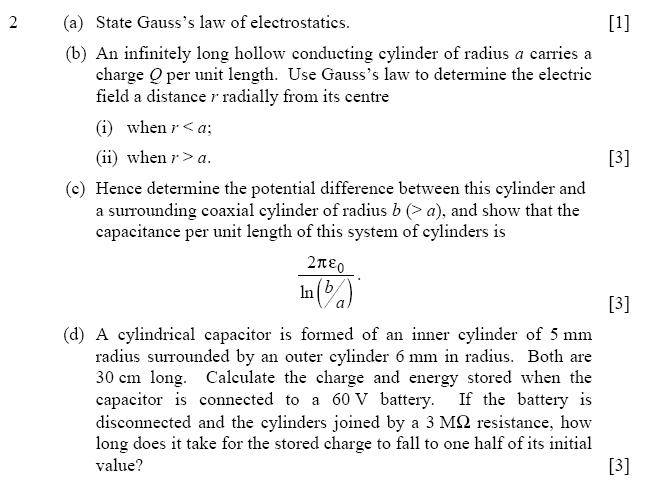# Using Gauss's Law to determine an electric field

## Homework Statement## Homework Equations

$${\phi} = \int E dA = \frac{QL}{\epsilon_0}$$

## The Attempt at a Solution

I'm aware of the definition of Gauss's law of electrostatics (however evidently I'm not very good at applying it), but I can't seem to fully answer the rest of the question.

An example in my book states the equation above and I've managed to simplify it to this state:

$$E = \frac{Q}{2\pi r \epsilon_0}$$

But I was wondering if this is the right route to go down, and if this is a correct equation, how I could use it to solve this problem.

vela
Staff Emeritus
Homework Helper
Looks good. You found the answer to (ii) in part (b). Do you know what the answer to (i) is?

answer to (i) (a) was given in your book or you know how to derive it?

Not quite sure how to derive it and I didn't even know I'd done the answer to part (ii) :X

Ok .. so you need to study applications of guass law

go here: http://hyperphysics.phy-astr.gsu.edu/hbase/electric/gaulaw.html" [Broken]

just study basics of usage of guass law ... and yes also please your questions's derivation too.

and come back on this forum when you have understood it ...